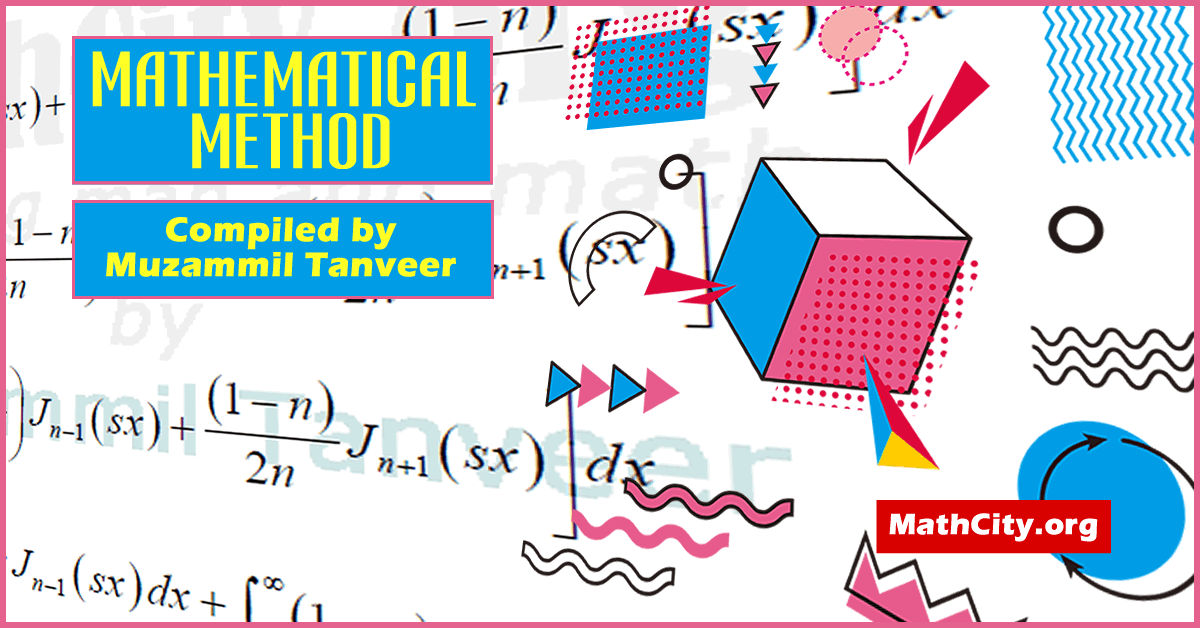# Mathematical Method by Sir Muhammad Awais AunMathematical methods are the approaches employed by mathematicians to address issues in mathematics and science. Algebra, functions, relations and associated graphs, calculus, and statistics are examples of mathematical techniques. Through their usage in resolving practical issues, they are applied to modelling.

Many physical occurrences are characterised by a function whose value at one place relies on its value at another. Derivatives of the function, such as the first derivative, which indicates the slope at a location, the second derivative, which indicates the curvature, and so forth, are included in the equation that determines this function. A differential equation is the name given to such an equation. Differential equations can describe a variety of common physical phenomena, including fluid movement, drum head vibrations, and heat dissipation in materials. A differential equation is one that contains or involves one or more derivatives of an unknown function. Differential equations can be divided into two categories. The first chapter of the notes is about differential equations. For other, please see what is in the notes?

These notes are provided and composed by Mr. Muzammil Tanveer. We are quite grateful to him for giving these notes, and we appreciate his efforts to make them available on MathCity.org.

• Name: Mathematical Method
• Lectured by: Sir Muhammad Awais Aun
• Provider: Mr. Muzammil Tanveer
• Pages: 117 pages
• Format: PDF
• Size: 1.1 MB
• Differential Equations
• Solution of a Differential Equation
• Linearly Independent Functions and the Wronskian
• Criterion for Linear Independent Functions
• Auxiliary Conditions
• Separated and Mixed Boundary Conditions
• Boundary Value Problems
• Abel’s Formula
• Eigenvalue Problems
• Self-Adjoint form of a Differential Equation
• Symmetric Operator
• Lagrange’s Identity
• Green’s Identity or Green’s formula
• Differential Equation
• Ordinary D.E (O.D.E)
• Partial D.E (P.D.E)
• Initial Condition
• Boundary Condition
• Fourier Transform
• Attenuation Property
• Even, Odd function
• Fourier Sine Transform
• Inverse Fourier
• Fourier Transformation Derivatives of a function
• Fourier Cosine Transformation
• Inverse Fourier Cosine Transformation
• Laplace Transformation
• Laplace transformation of derivative of function of f(t)
• Derivative of Laplace transformation
• Unit function
• Bessel Equation and Bessel Functions
• Basic Identities and Recurrence formula
• Parametric Bessel functions
• Transforming the independent variable for Bessel Function
• Orthogonality of Bessel functions
• Properties of Hankel transformation
• Hanker transformation of the derivation of the function f(x)
• Parseval Theorem
• Differential formula of Hankel transformation
• Green’s Identity or Green’s formula
• Green’s functions and their applications
• Green’s function for boundary value problems

Please click on View Online to see inside the PDF.

• notes/mathematical-method-muzammil-tanveer